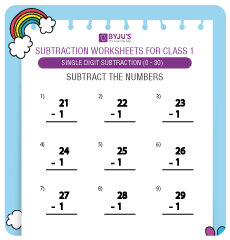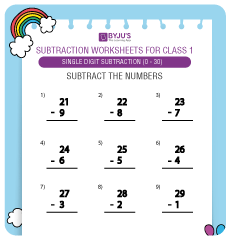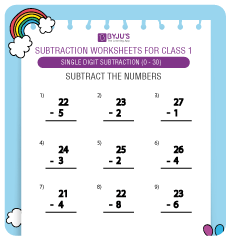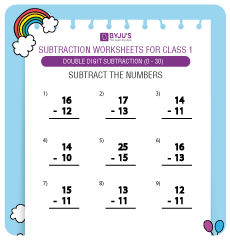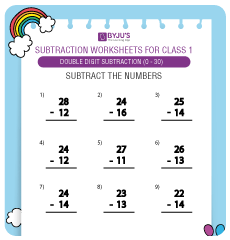# Double Digit Subtraction Worksheets for Grade 1 KidsHere we will discuss double digit subtraction and offer double digit subtraction worksheets for Grade 1 students. Subtraction is one of the four most important arithmetic operations which are taught to kids their formative years.

Double-digit subtraction is the subtraction of one double-digit number from another double-digit number. A good grasp of addition and subtraction is very crucial for kids. These are the basic operations. Addition and subtraction are learnt by kids prior to other two arithmetic operations- multiplication and division.

Given below are the 2 digit subtraction worksheets for your little one.

## 2 Digit Subtraction Worksheets for Grade 1 Kids (0 to 30):